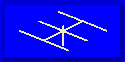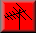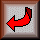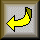##What Can We Expect From a 2-Element Beam?### L. B. Cebik, W4RNL### Method

A 2-element Yagi can be configured as either a director and driven element or as a driven element and reflector. We shall concentrate on the latter, because it is the most common configuration used.

My method will be modeling, using a variety of software: MININEC, NEC-2, and NEC-4. The advantage of the latter two engines is the availability of the Sommerfeld-Norton ground equations for more accurate modeling of antennas over real ground. However, some results will be cross-checked with MININEC in order to understand any differences that may arise. Models are convergence tested and use more than the minimum recommended number of segments per half-wavelength. Ground parameters will be average earth throughout.

All models will be for 10-meters, with a design center of 29 MHz. Although this frequency is well up the band from the region of greatest activity, it allows an examination of performance curves that cover maximum gain, maximum front-to-back ratio, and feedpoint impedance while trying to stay within the ham band.

The 10-meter models will employ single diameter aluminum elements. I have chosen 0.375" (3/8") elements because they scale well to other bands. The equivalencies from band to band for element diameter are as follows:

```     10 meters           3/8"
15                  9/16"
20                  3/4"
30                  1 1/8"
40                  1 1/2"```

In fact, all antennas for 10 meters noted below have been scaled for each of the ham bands mentioned, and--as expected--give identical performance figures.

Antenna heights will be given in fractions of a wavelength as well as feet. This procedure will permit more ready scaling of results to antennas designed for other bands. As a reference, the following table lists the heights of an antenna at 29 MHz in terms of both feet and fractions of a wavelength. Heights in feet are rounded to the nearest tenth of a foot.

```     1/8 wavelength       4.2'
1/4                  8.5'
3/8                 12.7'
1/2                 17.0'
5/8                 21.2'
3/4                 25.4'
7/8                 29.7'
1                   33.9'```

Scaling for lower ham bands by up to a factor of 10 should introduce no significant errors in results. Note that although heights of antennas for 10 meters would rarely be placed at a 4' level, antenna heights for the lowest amateur bands are often forced to corresponding low heights. An 80 meter dipole at 35' is very close to 1/8 wavelength above ground.

MININEC models of antennas at a height of 1/8 wavelength are not reliable, since the program only begins to approach reliability at antenna heights of 0.2 wavelength or greater. NEC with Sommerfeld-Norton ground implemented is reliable at the lower heights.

None of the models will press any of the other internal modeling limitations. All elements will be of a single diameter and linear, thus assuring easy convergence of results. Indeed, NEC-2 and NEC-4 should provide identical results, +/- 1 digit in the last decimal place of the output.

Models do have other limitations. Modeling programs assume level uniform terrain. Local variations can change some performance figures, such as the elevation angle of maximum radiation and feedpoint impedance. However, they do not change the basic expectation limits for any given design when related to other designs, since each design will be equally affected by the local terrain variations.

I shall look at the following types of 2-element Yagis: full-size, center- loaded with an inductor, center-loaded with a linear load, mid-element- loaded with an inductor, and capacity-hat loaded.

### Units of Measure

One of the most confusing aspects of antenna performance figures lies in the units of measure. Therefore, let me explain the units used here, working from the least confusing to the most confusing.

SWR: standing wave ratio. SWR, wherever apt to produce a curve, will be given relative to the resonated impedance of the test model at the design frequency. Hence, all antennas modeled will show close to 1:1 SWR at design center. Curves will assume that appropriate matching is employed, wherever apt, to the user's desired feedline impedance, with no examination of matching circuit losses. For certain models, a 50-ohm reference may also be used, since some common designs can be developed specifically for 50-ohm cable use.

Feedpoint Impedance (Z). Feed point impedance will always be given as a complex number involving resistance and reactance (R +/- jX) in ohms. Resonance will be defined as a reactance less than +/-1 ohm.

Front-to-back ratio (F-B). Front-to-back ratio will be given in dB below the maximum gain of the forward lobe. Front-to-back ratio is taken at a 180-degree angle from the forward lobe. One may also employ a notion of front-to-rear ratio, using the simple or complex mean of values in the quadrant extending for 180 degrees (or some other number of degrees) to the rear of the forward lobe. For the present enterprise, this procedure is unnecessary, since the rear lobe of a 2-element beam is usually geometrically simple. Hence, front-to-back ratio is a sufficient performance indicator for these tests.

Forward gain: The most complex question for the selection of units of measure is a proper characterization of gain. The most universal standard is gain in dBi, or dB over an isotropic source. Whenever two antennas require comparison, one simply subtracts on gain figure from the other to find the relative gain advantage or disadvantage.

A second common gain figure is dBd, dB relative to a dipole. However, when gain is registered in dBd, at least 2 different measures may be indicated:

dBdi: Gain in dBdi is gain relative to a free space dipole composed of lossless wire of infinitely thin diameter. This notion of dBd is easily related to the notion of dBi by the following equation: Gain dBi = Gain dBdi + 2.15. This notion of dBd has limited utility, but will be listed.

dBdr: Gain in dBdr is gain relative to a real dipole in the same defined situation as the test antenna. For modeling purposes, the dipole should be made from the same materials as the test antenna or from some predefined (and stated) set of materials. For these tests, the dipole will use the same material and element diameter as the test Yagi.

The notion of dBdr may also be used where gain figures result from range measurements. Whenever the notion is so employed, the complete set of test conditions for both the test antenna and the reference dipole should be explained. Otherwise, the figures cannot be meaningful, since replication of the test would not be possible. Range measurements will not be included in these notes. Therefore, dBdr will always refer to the gain of a full- length dipole of similar materials as the test antenna, and situated within the same parameters of height and ground specification.

However, a further complication arises in the use of dBdr. Even 2-element Yagi antennas exhibit for the same antenna height, a (usually slightly) lower elevation angle of maximum radiation than a dipole, especially for antenna height less than 1 wavelength. Therefore, simple gain figures must be accompanied by the elevation angle for those figures if a reasonable comparison is to be made.

### The Dipole Standard of Reference

Below is a table of gains for a full-size 3/8" aluminum dipole for various height from 1/8 to 1 wavelength. Gains are given in terms of dBi, dBdi, and dBdr to familiarize you with the differences in the gain recording systems. FS will always mean free space. Angle refers to the elevation angle of maximum radiation at which the gain figure is taken. Z is the feedpoint impedance given as R +/- jX ohms.
```Height    Gain in   dBi       dBdi      dBdr      Angle     Z
FS                  2.13      0         0         0         72 - j0.1
1/8                 4.60      2.45      0         87        55 - j0.0
1/4                 5.73      3.58      0         61        78 - j0.6
3/8                 6.07      3.92      0         37        85 - j0.7
1/2                 7.24      5.09      0         28        70 + j0.8
5/8                 7.75      5.60      0         22        64 + j0.2
3/4                 7.29      5.14      0         18        73 + j0.4
7/8                 7.15      5.00      0         16        78 + j0.6
1                   7.63      5.48      0         14        72 - j0.1
```

The required length of the dipole to achieve resonance varied from 16.0' to 16.4' but not in a linear progression. So too does the feedpoint impedance vary with height, but not in a pattern that corresponds to the variance in length. Gain also shows variations. From a peak at 5.8 wavelength, it drops to a low at 7/8 wavelength and climbs again to a peak at between 1 1/8 and 1 1/4 wavelengths. The variation is due to the amount of power contained in higher angle lobes.

It should be clear that, except in the most unusual circumstance, the concept of dBdi adds nothing to the analysis not already contained in the notion of dBi. For purposes of determining dBdr with respect to test antennas, we shall simply subtract the appropriate value of gain in dBi for the dipole from the value of gain in dBi for the test antenna at the same height.

Before we leave the subject of dBd, however, let's look at an all-too- typical claim. Suppose someone says that a certain antenna at a height of 7/8 wavelength has a gain of 5 dBd. How are we to understand this claim without further and full specification of what the idea of dBd means in this context? If we interpret the claim to mean dBdi, then the assertion is that the antenna has only the gain of a dipole, since a dipole at 7/8 wavelength has a gain of 5.00 dBi (assuming similar materials). If the antenna is an array, that would be a disappointing result.

If the claim is that the antenna has a gain of 5 dBdr (as applicable to modeling), then we would expect the antenna to have a gain of 12.15 dBi, since the gain of a dipole in dBi at 7/8 wavelength (assuming similar materials) is 7.15 dBi. Our next question is whether this claim is reasonable. To make such a judgment, we need to have some clear expectations of 2-element Yagi limits.Updated 5-5-97. © L. B. Cebik, W4RNL. Data may be used for personal purposes, but may not be reproduced for publication in print or any other medium without permission of the author.Go to Part 2: The Full-Size 2-Element YagiReturn to IndexGo to Amateur Radio Page